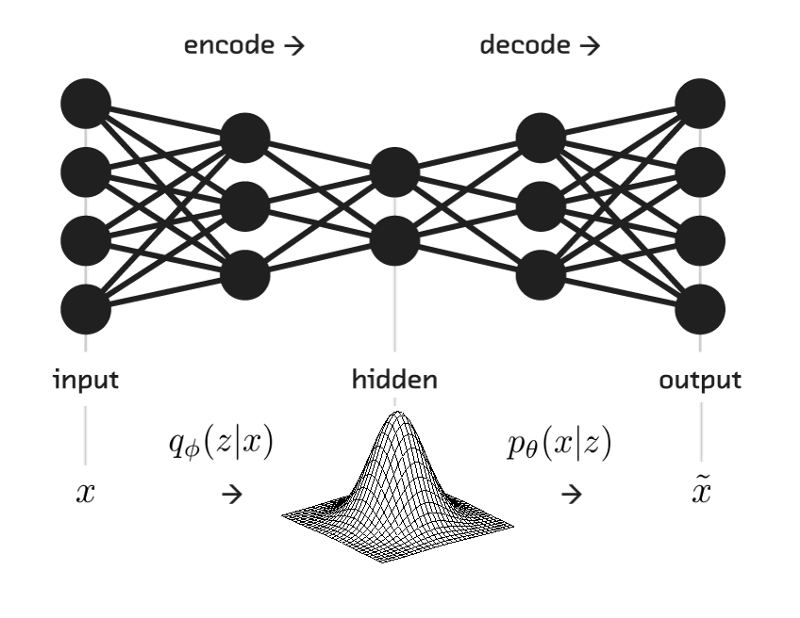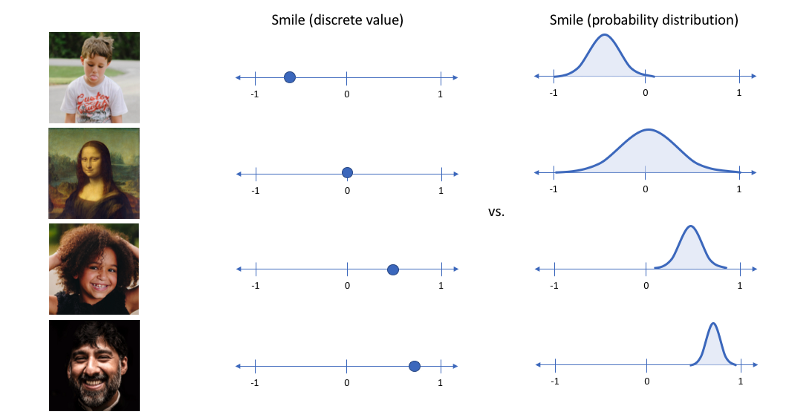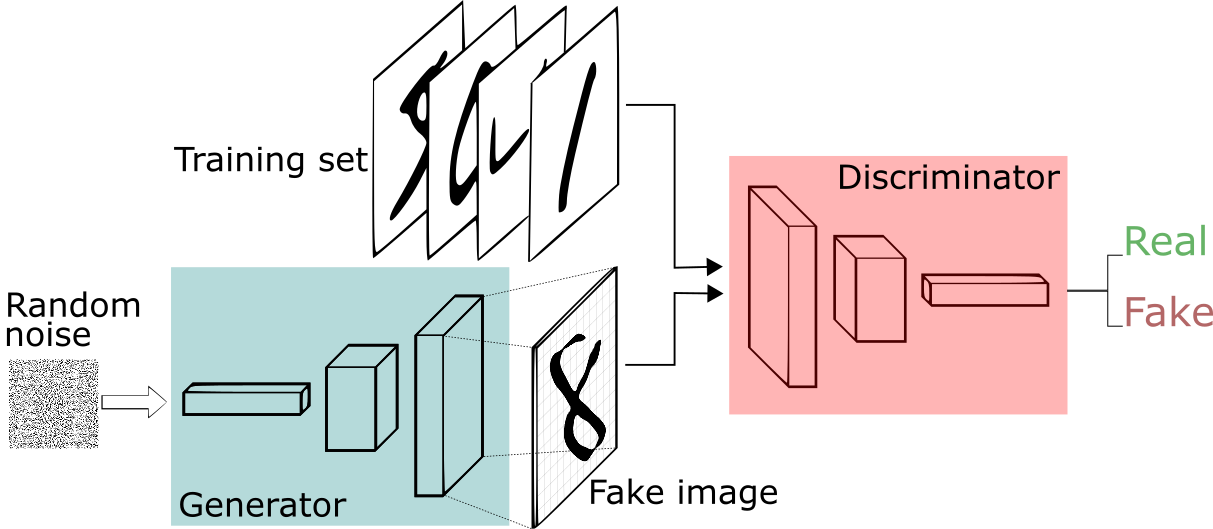×

Search anything:

# Generative Model

#### Machine Learning (ML) generative modelA Generative Model is a way of learning any kind of data distribution. It is used in unsupervised machine learning as a means to describe phenomena in data, enabling the computers to understand the real world.

In unsupervised machine learning, generative modeling algorithms process the training data and make reductions in the data. These models generally are run on neural networks and can come to naturally recognize the distinctive features of the data. The neural networks take these reduced fundamental understandings of real world data and then use them to model data that is similar or indistinguishable from real world data.

The main aim of all types of generative models is to learn the true data distribution of the training set so that the new data points are generated with some variations. But it is not possible for the model to learn the exact distribution of our data and so we model a distribution which is as similar to the true data distribution. For this, we use the knowledge of neural networks to learn a function which can approximate the model distribution to the true distribution.

### Different types of generative modeling:

The two types of generative models are:

1. Variational Autoencoder (VAE)

### Variational Autoencoder (VAE)

The definition of a VAE is that it “provides probabilistic descriptions of observations in latent spaces.” this means VAEs store latent attributes as probability distributions.

Let's first understand about the general architecure of VAE through the below diagram:The setup looks like the design of deep neural network and it consists of a pair of networks:

• the encoder
• the decoder

The encoder is responsible for the mapping of input x to posteriors distributions qθ(z∣x). similarly p(x∣z) is then parametrized by the decoder, combinely they form a generative network which takes latent variables z and parameters as inputs and projects them to data distributions pϕ(x∣z).

Each input image has features that can normally be described as single, discrete values. Variational autoencoders describe these values as probability distributions. Decoders can then sample randomly from the probability distributions for input vectors.A major drawback of VAEs is the blurry outputs that they generate.This is because data distributions are not recovered comletely.

Generative adversarial networks (GANs) are deep neural net architectures comprised of two nets, pitting one against the other (thus the “adversarial”).

GAN is having a huge scope or potential because they can learn to mimic any distribution of data. That is, GANs can be taught to learn anything in any domain: images, music, speech, prose. They are robot artists in a sense, and their output is impressive.

The general architecture of GAN is described below:One neural network, called the generator, generates new data instances, while the other, the discriminator, evaluates them for authenticity; i.e. the discriminator decides whether each instance of data it reviews belongs to the actual training dataset or not.

Let us assume we are going to generate hand-written numerals like those found in the MNIST dataset, which is taken from the real world. The goal of the discriminator, when shown an instance from the true MNIST dataset, is to recognize them as authentic.

Meanwhile, the generator creates images and passes it to the discriminator. The goal of the generator is to generate passable hand-written digits, to lie without being caught. The goal of the discriminator is to identify images coming from the generator as fake.

Here are the steps a GAN takes:

• The generator takes random numbers and returns an image.
• This generated image is fed into the discriminator alongside a stream of images taken from the actual dataset.
• The discriminator takes in both real and fake images and returns probabilities, a number between 0 and 1, with 1 representing a prediction of authenticity and 0 representing fake.#### Prashant Anand

Bachelor of Technology (2016 to 2020) in Electronics and Communications Engineering at Reva University, Bangalore | Intern at OpenGenus